# What Are Trig Functions Used For? (12 Real Life Examples)

We learn about trig functions such as sine, cosine, and tangent in high school math courses.  However, this often leads students to wonder how these ideas are applied in technology and other disciplines.

So, what are trig functions used for in real life?  Trig functions are used or found in architecture & construction, communications, day length, electrical engineering, flight, GPS, graphics, land surveying & cartography, music, tides, optics, and trajectories.

Of course, the trig function we use will depend on the situation we find ourselves in.  Often, a computer will perform the calculations, but the mathematical concepts are still very much necessary for our technology to work correctly.

In this article, we’ll take a closer look at 12 real life examples of how trig functions are used every day.

Let’s get started (You can watch a video version of this article on YouTube).

## What Are Trig Functions Used For? (12 Real Life Examples)

Trig functions are used in real life a lot more often than you think.  Although you might not need to use trig functions in your work, they probably shows up many times throughout your day – without you even realizing it!

(If it’s been a while, you can get a quick refresher on trig functions with this resource from Clark University).

Here are some fields that use trigonometric functions:

Uses Of Trig Functions
Uses Of Trig Functions
• Architecture & Construction
• Communications
• Day Length
• Electrical Engineering
• Flight
• GPS
• Graphics
• Land Surveying & Cartography
• Music
• Tides
• Optics
• Trajectories

### Architecture & Construction

Trig functions are used for architecture and construction in many different ways.  Some of the uses include:

• Building Height
• Pitch of a Roof
• Trusses & Supports

Dartmouth College has an interesting article about how an old method to find the height of a point on a building is still used to this day.

We can also use tangent to calculate the height of the roof of a building, given the width of the roof and the desired angle (or “pitch”) of the roof.

If H is the height, W is the width, and A is the angle (pitch) of the roof, then we can use the equation:

• tan(A) = H / W

since tangent is opposite (height) divided by hypotenuse.  We can rearrange (by multiplying W on both sides) to get:

• H = Wtan(A)

So, to find the height of the roof, we take the tangent of the angle (pitch) of the roof and then multiply by the width of the roof.

So, if we wanted a pitch of 45 degrees (fairly steep!) for a roof that is 40 feet wide, we would have A = 45 degrees and W = 40 feet.

We could then calculate the height of the roof using our formula:

• H = Wtan(A)  [original formula]
• H = 40tan(45)  [substitute H = 40 feet and W = 45 degrees]
• H = 40 [since tan(45) = 1]

So, the height of the roof would also be 40 feet.

In architecture, weight plays a huge role in how we construct building.  More weight means more force is exerted, which means we need more support for a heavy roof or bridge.

Architects can use trig functions to find the horizontal (x) and vertical (y) force components to figure out how to build a truss to support a bridge.  Proper calculation of force components (using trig!) prevents the bridge from collapsing under its own weight, leaning to one side, or buckling due to imbalanced forces.

### Communications

Trig functions are also used in communications, such as radio waves and other signals.  A radio wave can be described as sinusoidal, since it repeats periodically (just like a sine function) and has the same pattern over time.

A sinusoidal function f(x) = Asin(Bx) can describe a radio wave.

The amplitude, A, tells us how “tall” the wave is.  A larger amplitude means a taller wave.

This is the same A as in AM radio (amplitude modulation).  When you change the amplitude, you change the shape of the wave and the signal that is sent and received.

The period, 2*pi / B, tells us how long it takes the wave to go through one full “cycle”.  When you change the amplitude, you change the shape of the wave and the signal.

The frequency, F = 1 / period or F = B / 2*pi, tells us how often the wave repeats.  A higher frequency means a faster moving wave.

This is the same F as in FM radio (frequency modulation).  When you change the frequency, you change the speed of the wave and the signal that is sent and received.

For example, the sine function f(x) = 2sin(4x) has twice the amplitude and 4 times higher frequency than g(x) = sin(x).

### Day Length

The day length (from sunrise to sunset) varies throughout the year, changing by a small amount each day.  In fact, the amount that day length increases or decreases is not the same every day!Day length (time from sunrise to sunset) varies by the seasons. We can model the day length with a sine function.

The reason is that we can model day length with a sinusoidal function.  This means that day length has a maximum and a minimum, and it increases or decreases at different rates during the year.

On the other hand, day length changes a lot each day near the “midpoints” between the shortest and longest days: we gain or lose about 3 minutes per day around March 21 and September 21.

Of course, the change in day length also varies depending on latitude (how far north or south you are from the equator).

### Electrical Engineering

Trig functions also appear in electrical engineering.  For example, the Impedance Triangle relates the Resistance (R), Reactance (X), and Impedance (Z) of a circuit.

We can use a trig function to find the angle between R and X, depending on which values R, X, and Z we know.

### Flight

Trig functions are useful in flight to determine the course a plane should take to arrive at its destination.  Of course, the following factors must all be taken into account:

• plane’s propulsion
• plane’s weight (which changes during the flight as fuel is burned!)
• wind speed
• wind direction

We would need to use vectors and trig functions to determine the angle a plane should fly at to maintain course.  However, this will happen more than once during a flight.Trig functions are used to make course corrections to help keep an airplane on the right path.

In fact, small course corrections are made throughout a flight.  The reason is that the wind can change often, especially during a long flight over a great distance.

### GPS

GPS (global positioning system) uses trigonometry as part of a triangulation to find the position of an object on Earth (triangulation involves the Law of Sines).GPS uses trig (in triangulation) to find out where you are and helps you get to where you are going.

### Graphics

Trig functions are also used often in computer graphics to determine the position of an object based on its speed (and its speed based on its acceleration).

This is useful in video games, movies, and other applications.  As in architecture, trig functions are used to break up a vector (velocity or acceleration) into its components (x and y directions, or possibly even z directions in 3D applications).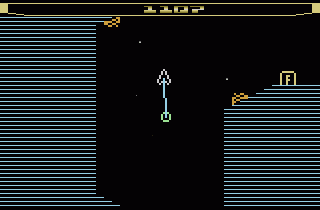Trig functions help to figure out where an object is going to appear on the screen, based on its current position, velocity, and acceleration.

For example, let’s say an object on-screen is traveling at a speed of 50 miles per hour and moving northeast at 30 degrees from the horizontal.

Then the speed components are:

• Horizontal Speed: +50cos(30), or about 43.3 miles per hour east.
• Vertical Speed: +50sin(30), or 25 miles per hour north.

We can use these units to move the object the proper distance north and east on the screen using pixels (the smallest unit in a graphics system, which indicates screen size).

### Land Surveying & Cartography

Trigonometry also appears in land surveying and cartography (map making).  For example, let’s say we want to find the distance across a river at a certain point.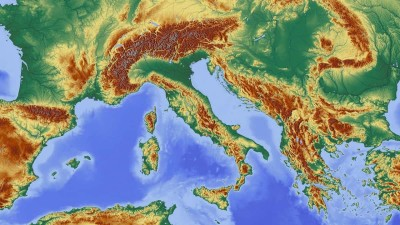Trig functions can be used to find distances for map making.

First, we mark our starting position.  Next, we identify a stationary object across the river.

Then, we mark a line between our starting position and the stationary object across the river.  The next step is to move a known distance along a line perpendicular to the line across the river.

Finally, we find the angle between the marker at the starting position and the stationary object across the river.

Since we moved a known distance along a perpendicular line, we have a right triangle.  We also know one of the side lengths (the distance we moved) and one of the angles.

We can then use a trig function (tangent) to find the length of the other leg of the triangle (the distance across the river).

The equation would be:

• tan(x) = D / K

rearranging, we get:

• D = Ktan(x)

where x is the angle between the starting position and the stationary object, D is the distance across the river, and K is the known distance we walked from the starting point.

### Music

Trig functions are also involved in music, although not in an obvious way.  We can model a sound with a sine wave:

• The frequency corresponds to the pitch (high frequency = high note)
• The volume (loudness) corresponds to the amplitude of pressure (high pressure = high volume)

When you hear (or play) music, you have something that can be expressed as the sum of these various sine waves (this result is due to Joseph Fourier).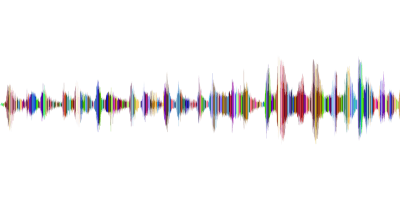We can express a sound wave as a sum of various sine waves.

### Tides

Similar to day length, we can also model tides using trig functions.  Tides vary between low tide and high tide about every 6 hours (this means that the period is about 12 hours).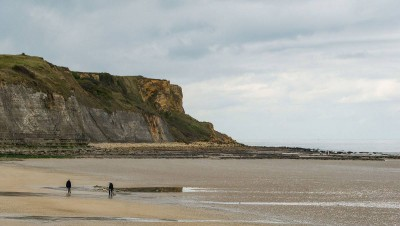At low tide, you can see how much higher the water can get!

The tide (which can be high, low, or somewhere in between) varies throughout the day, changing by a small amount each minute.  In fact, the amount that the tide increases or decreases is not the same every minute!

The reason is that we can model tide with a sinusoidal function.  This means that the tide has a maximum and a minimum, and it increases or decreases at different rates during the day.

On the other hand, the tide changes a lot each day near the “midpoints” between the low tide and high tide: the tide changes the most at the 3 hour mark between low and high tide, or around 12:40PM, 6:40PM, 12:40AM, and 6:40AM).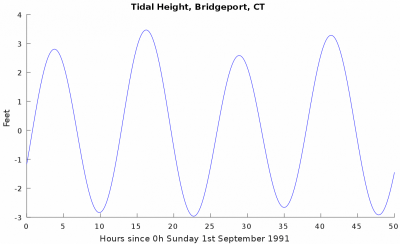A tide graph show a picture that looks like a sinusoidal function.

Of course, the change in tide also varies depending on location (how far north or south you are from the equator).

### Optics

Trig functions can help us in the field of optics. For example, we can figure out how light travels via reflection and refraction.

In particular, the law of refraction involves the sine function. Of course, the material that the light is traveling through will affect how fast it travels (known as the material’s refractive index).

### Trajectories

Trig functions are used all the time in any discipline that requires us to analyze trajectories, including:

• Ballistics (useful for crime scene investigation or firearms manufacturers)
• Weapons (useful for calculating trajectories, speed, acceleration, and angles necessary to intercept another object, as in missile defense systems).

As with so many other disciplines, trig functions are used to break down a trajectory into components (x and y, and possibly a z component).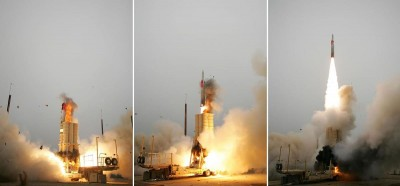Trig functions are used to calculate trajectories in missile defense systems.

## Conclusion

Now you know all about how trig functions are used in real life.  The applications are astounding in their scope, and they make our lives much better and more enjoyable, don’t you think?

Check out my article on trig functions and how to figure out their signs, based on the quadrant.

You might also want to read my article on the four quadrants and what they mean.

I hope you found this article helpful.  If so, please share it with someone who can use the information.

~Jonathon